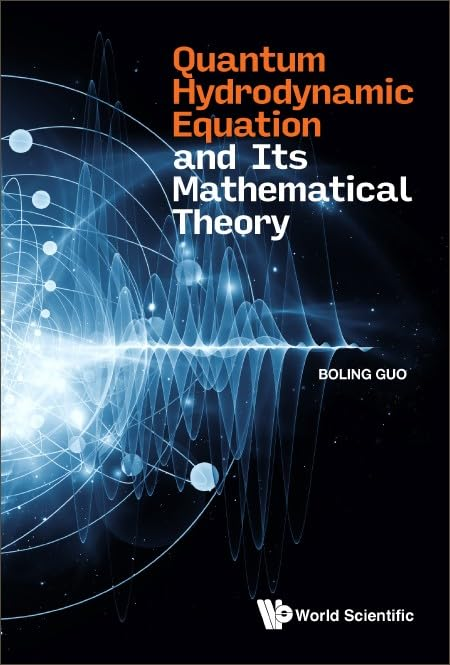# Quantum Hydrodynamic Equation And Its Mathematical Theory

• Length: 320 pages
• Edition: 1
• Publisher:
• Publication Date: 2023-06-22
• ISBN-10: 9811260834
• ISBN-13: 9789811260834
Description

Quantum hydrodynamics comes from superfluid, superconductivity, semiconductor and so on. Quantum hydrodynamic model describes Helium II superfluid, Bose–Einstein condensation in inert gas, dissipative perturbation of Hamilton–Jacobi system, amplitude and dissipative perturbation of Eikonal quantum wave and so on. Owing to the broad application of quantum hydrodynamic equations, the study of the quantum hydrodynamic equations has aroused the concern of more and more scholars. Based on the above facts, we collected and collated the data of quantum hydrodynamic equations, and studied the concerning mathematical problems. The main contents of this book the derivation and mathematical models of quantum hydrodynamic equations, global existence of weak solutions to the compressible quantum hydrodynamic equations, existence of finite energy weak solutions of inviscid quantum hydrodynamic equations, non-isentropic quantum Navier-Stokes equations with cold pressure, boundary problem of compressible quantum Euler-Poisson equations, asymptotic limit to the bipolar quantum hydrodynamic equations.